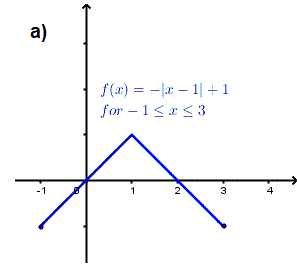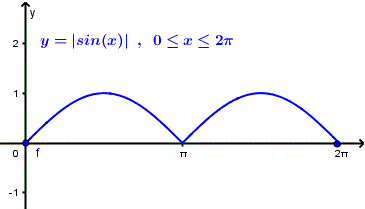# Rolle's Theorem Questions and Examples

Rolle's theorem is a special case of the mean value theorem. It is discussed here through examples and questions.

## Rolle's Theorem

Rolle's theorem is the result of the mean value theorem where under the conditions:
f(x) be a continuous functions on the interval [a, b]
and differentiable on the open interval (a, b) , there exists at least one value c of x such that f '(c) = [ f(b) - f(a) ] /(b - a).
Now if the condition f(a) = f(b) is satisfied, then the above simplifies to : f '(c) = 0.
In other words, under the above three conditions we can always find a tangent to the curve of f that is horizontal (slope = f'(c) = 0).
Rolle's Theorem
If f(x) is
1) a continuous function on the interval [a, b]
2) differentiable on the open interval (a, b)
3) and f(a) = f(b),
then there is at least one value c of x in the interval (a, b) such that
f '(c) = 0

## Example 1

The graph of f(x) = - x2 + 6x - 6 for 1 ? x ? 5 is shown below. f(1) = f(5) = - 1 and f is continuous on [1 , 5] and differentiable on (1 , 5) hence, according to Rolle's theorem, there exists at least one value of x = c such that f '(c) = 0.
f '(x) = - 2 x + 6
f '(c) = - 2 c + 6 = 0
Solve the above equation to obtain
c = 3
Therefore at x = 3 there is a tangent to the graph of f that has a slope equal to zero (horizontal line) as shown in figure 1 below.

## Example 2

The graph of f(x) = sin(x) + 2 for 0 ? x ? 2? is shown below. f(0) = f(2?) = 2 and f is continuous on [0 , 2?] and differentiable on (0 , 2?) hence, according to Rolle's theorem, there exists at least one value (there may be more than one!) of x = c such that f '(c) = 0.
f '(x) = cos(x)
f '(c) = cos(c) = 0
The above equation has two solutions on the interval [0 , 2?]
c1 = ?/2 and c2 = 3?/2.
Therefore both at x = ?/2 and x = 3 ?/2 there are tangents to the graph that have a slope equal to zero (horizontal line) as shown in figure 2 below.

## Example 3

Function f in figure 3 does not satisfy Rolle's theorem: although it is continuous and f(-1) = f(3), the function is not differentiable at x = 1 and therefore f '(c) = 0 with c in the interval (-1 , 3) is not guaranteed. In fact it is easy to see that there is no horizontal tangent to the graph of f on the interval (-1 , 3).Figure 3. Graph of function that does not satisfy the condition of differentiability in Rolle's theorem

## Question 1

Which of the functions given below satisfy all three conditions of Rolle's theorem?
a) f(x) = cos(x) , for x in [0 , 2?]
b) g(x) = |x - 2| , for x in [0 , 4]
c) h(x) = 1 / x2 , for x in [-1 , 1]
d) k(x) = |sin(x)| , for x in [0 , 2?]

### solution to question 1

a)
f(0) = 1 and f(2?) = 1 therefore f(0) = f(2?)
f is continuous on [0 , 2?]
Function f is differentiable in (0 , 2?)
Function f satisfies all conditions of Rolle's theorem
b)
function g has a V-shaped graph with vertex at x = 2 and is therefore not differentiable at x = 2.
Function g does not satisfy all conditions of Rolle's theorem
c)
Function h is undefined at x = 0.
Function h does not satisfy all conditions of Rolle's theorem.
d)
k(x) = |sin(x)| , for x in [0 , 2?]
The graph of function k is shown below and it shows that function k is not differentiable at x = ?.
Function k does not satisfy all conditions of Rolle's theorem.Figure 4. Graph of k(x) = |sin(x)| , for x in [0 , 2?]

## Question 2

Check that function f(x) = x 2 - 4 x + 3 on the interval [1 , 3] satisfies all conditions of Rolle's theorem and then find all values of x = c such that f '(c) = 0.

### solution to question 2

f is a polynomial function and is therefore continuous on the interval [1 , 3] and differentiable on the interval (1 , 3). Also f(1) = f(3) = 0 and therefore function f satisfies all three conditions of Rolle's theorem and there is at least one value of x = c such that f '(c) = 0.
f '(x) = 2 x - 4
f '(c) = 2 c - 4 = 0
Solve for c to obtain
c = 2.
The graph below shows f , f ' and the tangent at x = c = 2 is horizontal; and f '(2) = 0Figure 5. Rolle's theorem , question 2, f(x) = x 2 - 4 x + 3

## Question 3

Check that function g(x) = cos(x) on the interval [- ?/2 , 3?/2] satisfies all conditions of Rolle's theorem and then find all values x = c such that g '(c) = 0.

### solution to question 3

Function g is a cosine function and is therefore continuous on the interval [- ?/2 , 3?/2] and differentiable on the interval
(-?/2 , 3?/2). Also g(- ?/2) = g(3?/2) = 0 and therefore function g satisfies all three conditions of Rolle's theorem and there is at least one value of x = c such that f '(c) = 0.
g '(x) = - sin(x)
g '(c) = - sin(c) = 0
Solve for c to obtain
c = n? , n = 0,Ý 1 , Ý 2 , ...
Solutions on the interval [- ?/2 , 3?/2] are
c1 = 0 and c2 = ?
The graph below shows g , g ' and the tangents at x = c1 = 0 and x = c2 = ? are horizontal; and g '(0) = 0 and g '(?) = 0Figure 5. Rolle's theorem , question 3, g(x) = cos(x)